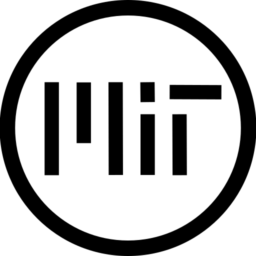# Goop Projectile

Purpose

A Godot 3.5 goop-like object to serve as a viscous projectile in this game.

Highlights

• Lerp between two colors
• Direction of animation can be set via code

How to Get Started

Create a new sprite with an image such as the one shown as screenshot 1.

Under shader params, create new NoiseTexture for the Flow Map parameter. Under noise, create new Simplex noise. See screenshot 2 for suggested values.

How to Set Direction

This code can be placed in the Sprite parent and shows how the shader parameters can be set with code. Note that setting strength to a random number is optional. Line 3 is required if each instance of the projectile is to have its own unique look. “Push_direction” Should be a Vector2 and relate to the direction the projectile will be moving in.

`    var s : Sprite = get_child(0)`
`    var mat : ShaderMaterial = s.material`
`    s.material = mat.duplicate()`
`    mat.set_shader_param("direction", push_direction)`
`    mat.set_shader_param("strength", rand_range(0.2,0.9))`

``````// Written in part by Erich_L, MIT and free to use <3

// Displacement map (set this manually in editor)
uniform sampler2D flowMap;

// Strength of the displacement effect
uniform float strength = 0.3;

// Direction of displacement flow (set this with GDscript)
uniform vec2 direction = vec2(1.0, 1.0);

// Two colors to interpolate between (noise based)
uniform vec4 color1 : hint_color = vec4(0.007843, 1.0, 0.972549, 1.0);
uniform vec4 color2 : hint_color = vec4(1.0, 1.0, 1.0, 1.0);

void fragment(){
vec4 noise_sample = texture(flowMap, vec2(UV.x + (TIME * direction.x * -1.0), UV.y + (TIME * direction.y))); // Sample the noise
vec4 offset = noise_sample * strength; // Apply strength scalar for a UV offset
vec4 texture_check = texture(TEXTURE, vec2(UV.x,UV.y) + offset.xy - vec2(0.5,0.5)*strength); // Sample the texture using the UV offset
vec4 color = mix(color1, color2, noise_sample.x * 1.3); // Sample the two colors
COLOR = vec4(color.xyz, texture_check.a); // Define final output, using the alpha of the original image
}``````
###### Tags
bullet, displacement, Goop, ooze, projectile, viscousThe shader code and all code snippets in this post are under MIT license and can be used freely. Images and videos, and assets depicted in those, do not fall under this license. For more info, see our License terms.

## More from Erich_L

### “Unit Selected” Oscillating Circle

Subscribe
Notify of